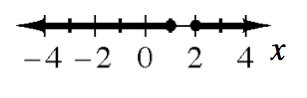### Home > INT3 > Chapter 6 > Lesson 6.2.2 > Problem6-50

6-50.

Solve the following inequalities. Represent each solution on a number line.

1. $x^2 - 2x < 3$

Change the inequality into an equation and solve for $x$ to find the boundary points. Plot the boundary points on a number line. Test values of $x$ to find where the inequality is true. Shade the true region and describe it with one or more inequality statements.

1. $3x-x^2\le2$